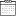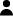# A mixed control problem of the management of natural resources

• Home
• A mixed control problem of the management of natural resources7 December 2015By CEE-M

In this paper, we study a mixed optimal control problem in which both continuous controls and impulse controls are admissible. The optimal solution of this problem can be characterized via the Hamilton-Jacobi-Bellman equation. However, the resolution of this equation can be cumbersome and in most cases only numerical solutions are possible. This is why we consider an example with particular functional forms. Building on the solutions of the pure continuous control and the pure impulse control problem, we propose a candidate for the optimal solution of the mixed control problem. We prove that this candidate verifies the Hamilton-Jacobi-Bellman equation. As conjectured by Clark, jumping to the steady state and then staying there is one possible optimal solution, but we show that the profit functions of the continuous control and impulse control sub-models need to be related in a particular manner to reach such solution. Although the above results were obtained with particular functional forms, we are working to prove the optimality of Clark’s policy for general functional forms, maintaining the relationship between the profit functions